Disclosure: This post may contain affiliate links, meaning when you click the links and make a purchase, we receive a commission.

# How to calculate compound interest for recurring deposit in Excel!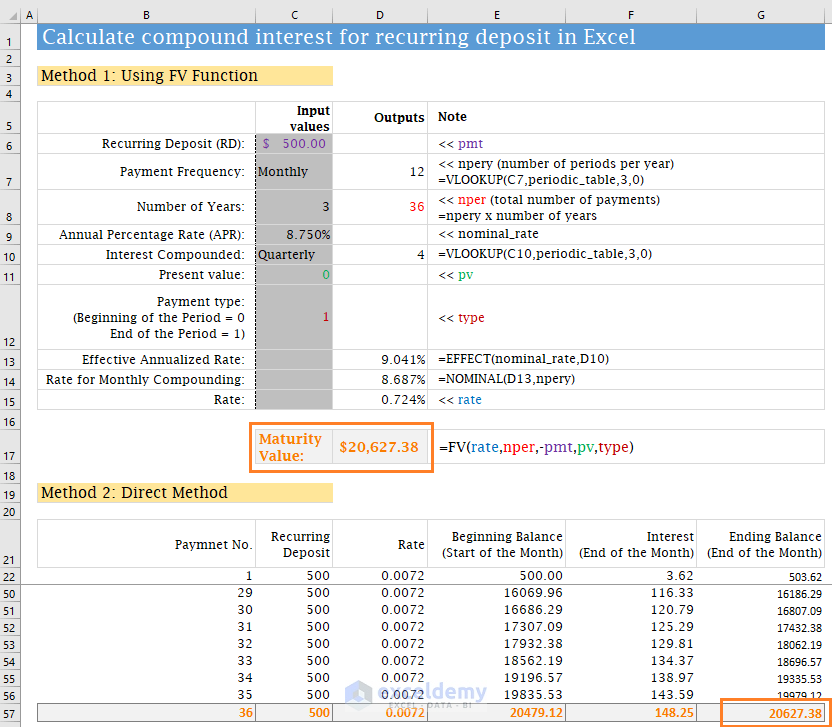In this short tutorial, I will show how to calculate compound interest (maturity value) for recurring deposits in Excel.

If you don’t have a tight budget, the saving will be tough for you. But saving is important in life for so many reasons. We want to save:

• For our emergency fund
• For making our life debt-free
• For our retirement
• For our children education
• And for building wealth

“Do not save what is left after spending, but spend what is left after saving.” – Warren Buffett

For some people, saving is tough. For these types of people, making a recurring deposit every month to their savings account and getting a good amount after some years is a good option.

This Recurring Deposit (RD) is a popular saving scheme in the Indian sub-continent. I am from Bangladesh and a recurring deposit (RD) is a very popular scheme in my country, too.

Recurring Deposit (RD) is popular for the following reasons:

• Investors can save a small amount of money every month
• This scheme forces them to save every month
• Safe and assured return of their investments
• Income tax is lower or nil in this scheme

Let me now show you how you can calculate your maturity value of the recurring deposits in Excel. It is a good practice to know how the whole thing works of your investment. Never be in the dark when this is about your personal finance.

Calculate-compound-interest-for-recurring-deposit.xlsx

## Calculate compound interest for recurring deposit in Excel

Excel has made our life very easier. Using Excel’s FV function, you can easily calculate the Maturity Value (Future Value) of your recurring deposit for any periods.

Complexity arises when you make deposits monthly but the Bank compounds your money quarterly or in different periods. Don’t worry. I will make things easier for you.

I will explain the whole calculation step by step.

Some Excel functions, we shall use:

1) FV Function

FV function returns the future value of an investment based on periodic, constant payments and a constant interest rate.

Syntax of FV function: FV(rate, nper, pmt, pv, type)

Here,

• rate: The Interest rate per period
• nper: Total number of periods
• pmt: The payment made in each period
• pv: Present value
• type: Type of payment. Payments can be of two types: the Beginning of the period (1) and End of the period (omitted or 0).

2) EFFECT Function

EFFECT function returns the effective annual interest rate.

Syntax of EFFECT Function: EFFECT(nominal_rate, npery)

Here,

• nominal_rate: Nominal Annual Interest Rate
• npery: Number of compounding will happen in a year

For example, your bank provides the Nominal Interest Rate is 6% per annum. Now you make a deposit of amount \$100 with a bank for the next 1 year and the bank compounds your money quarterly.

What will be your effective rate or return?

Your Rate per Quarter is: 6%/4 = 1.50%. This is because your money is compounded 4 times per year. So, nominal interest is divided by 4 to get the Rate per Quarter.

Look at the image below.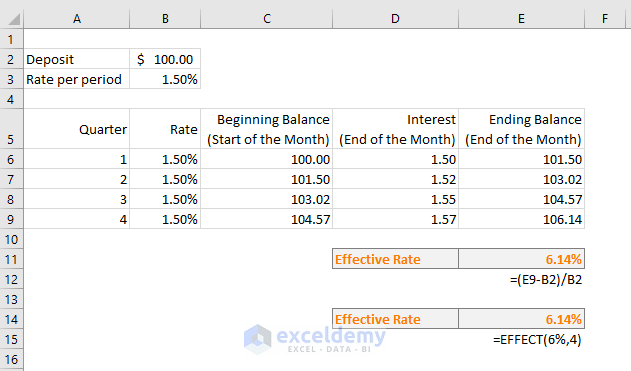You see that:

• At the end of the first quarter, your ending balance will be \$101.50. 1.50% interest is applied to the Beginning Balance \$100.
• 50 is the beginning balance at the start of the 2nd quarter. At the end of the 2nd quarter, your ending balance will be \$103.02. 1.50% interest is applied on the beginning balance of \$101.50
• At the end of the fourth quarter, your ending balance will be: \$106.14

Your nominal interest rate is 6%. But because of 4 compoundings per year, you’re getting a 6.14% return on your investment.

We can use the EFFECT function to get the above Effective Rate:

=EFFECT(6%,4)

This is shown also in the image.

3) NOMINAL Function

The NOMINAL function is the opposite of the EFFECT function. It returns the Nominal Interest Rate from an Effective Interest Rate.

Syntax of NOMINAL Function: NOMINAL (effect_rate, npery)

=NOMINAL(6.14%,4)

= 6.00%

### Method 1: Using Excel’s FV Function

The following image shows the whole process that I have used to calculate the recurring deposit. In this method, I have used the FV function.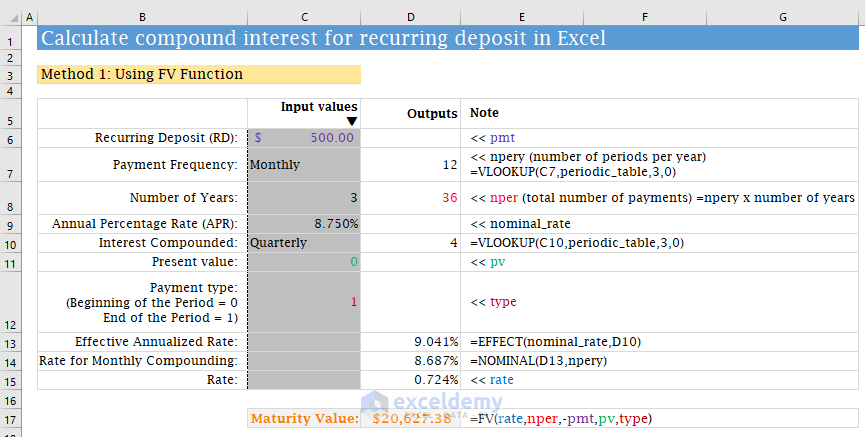Explanation of the above image:

You see there is a column for input values. You will input the following values:

• Recurring Deposit (RD): The amount you will deposit every month (or any period). We named this cell pmt.
• Payment Frequency: It is a drop-down list. In most cases, it is monthly. But you can select any period from the drop-down.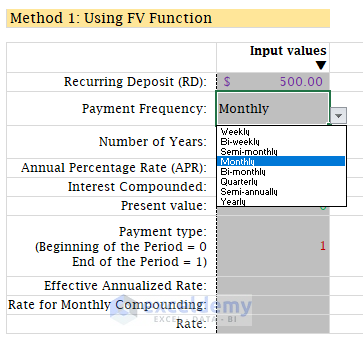On the right, we have also calculated the number of periods per year (npery) using Excel’s VLOOKUP function: =VLOOKUP(C7,periodic_table,3,0). You will get the periodic_table in the Tables worksheet. I have shown the periodic_table in the following table.

 Interest Compounded Calculated After (Days or Months) No. of Payments/Year Weekly 7 52 Bi-weekly 14 26 Semi-monthly 15 24 Monthly 1 12 Bi-monthly 2 6 Quarterly 3 4 Semi-annually 6 2 Yearly 12 1

• Number of Years: Total number of years you will keep depositing your fund. On the right, we get the total number of periods (nper) multiplying the Number of Years by the Number of Periods per Year (npery).
• Annual Percentage Rate (APR): This is the nominal interest rate your bank offers to you.
• Interest Compounded: Indian Banks compound your investment quarterly. It can differ from bank to bank. This is also a drop-down list. So, you can choose any compounding frequency.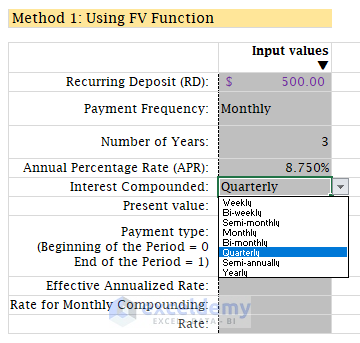Just remember that your Interest Compounding Frequency must be equal to or greater than the Payment Frequency. For example, if your Payment Frequency is Monthly, you cannot choose the Compounding Frequency value as Weekly, Bi-weekly, or Semi-monthly.

On the right side, you’re seeing the Number of Compounding per Year. We got it using the VLOOKUP Excel function: =VLOOKUP(C10,periodic_table,3,0)

• Then you will input the Present Value, and Payment Type (either 0 or 1).

Now the Most Complex Part:

Our Recurring Deposit is made Monthly but our compounding frequency is Quarterly (or any).

So how will we handle this situation?

Think with me:

• What we need is the same yearly effective rate. So, we shall calculate the effective rate for Quarterly compounding: =EFFECT(8.75%,4) = 041%
• Now we need a nominal rate that will give us the same effective rate with Monthly compounding: =NOMINAL(9.041%,12) = 8.687%. You can cross-check it this way: whether this nominal rate (8.687%) will provide the same effective rate with monthly compounding: =EFFECT(8.687%,12) = 041%. Same.
• Now we need the rate for a period (monthly) and it is 687%/12 = 0.724%.

Now the rest is simple. I have used the FV function in the cell D17 to the maturity value. The result is: \$20,627.38

### Method 2: Direct Method

This is a step by step calculation to get the Maturity Value of your Recurring Deposit (RD) for 36 periods (3 years).

We have used the same pmt value, the same rate per period here. But the procedure is direct. Let me explain.

See the image below.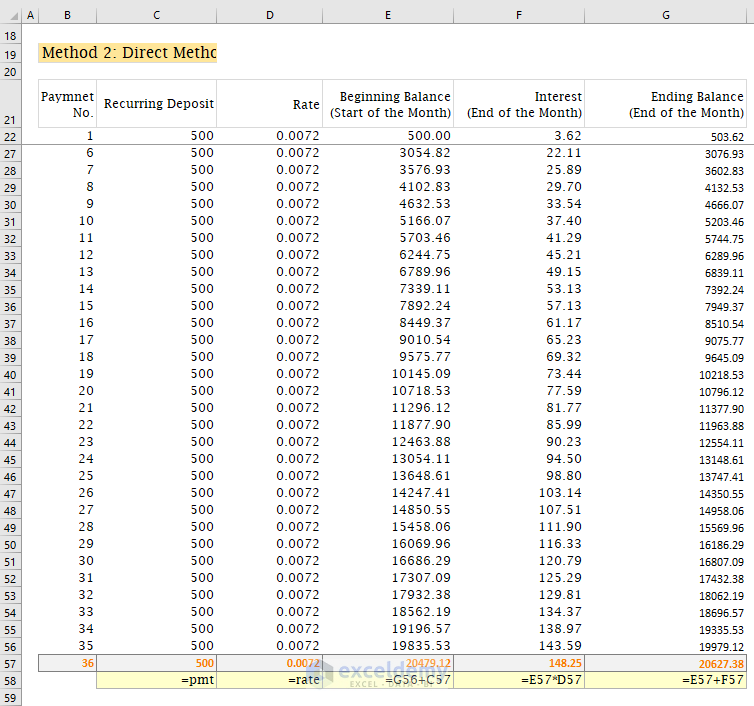Explanation of the image:

• At the start of the month, you deposit \$500. At the end of the month, you get an interest in the amount of \$3.62. So, your ending balance = \$500 + \$3.62 = 503.62
• At the start of the second month, you deposit another \$500 and your previous month ending balance was \$503.62. So, at the start of the 2nd month, your total Beginning Balance is \$503.62 + \$500 = \$003.62. You get the interest in the amount of \$7.27 on this amount (\$1003.62). So, at the end of the 2nd month, your ending balance will be: \$1003.62 + \$7.27 = 1010.88
• This is how the table advances.
• To add more periods in this table, just copy the last row to your desired level.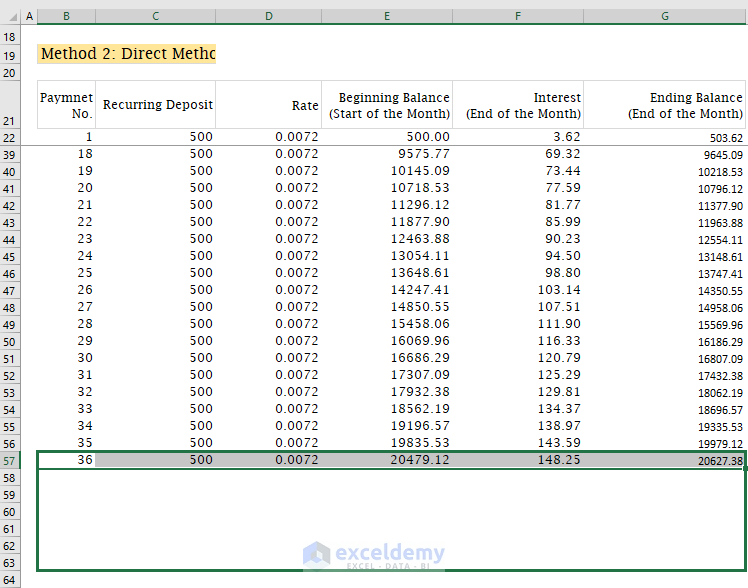## Conclusion

If you have followed this article step by step, you have learned a very important lesson. Before using Excel, I struggled a lot to calculate even my wife’s recurring deposits and its Maturity Value. Now it is simple to me.

So, this is my way of calculating interest for recurring deposits in Excel. What is your feedback with this article? Was it useful? Did you face any problem using my calculator? Let me know in the comment box.

Hello!
Welcome to my Excel blog! It took me some time to be a fan of Excel. But now I am a die-hard fan of MS Excel. I learn new ways of doing things with Excel and share here. Not only how to guide on Excel, but you will get also topics on Finance, Statistics, Data Analysis, and BI. Stay tuned!

1 Comment
1. ReplySurya Aug 18, 2019 at 11:54 PM

Thank you for this useful article. The maturity does tally with the Indian banks (e.g. Axis Bank) as calculated in the Bank’s website.